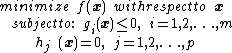# nonlinear programming

Also found in: Acronyms, Wikipedia.

## nonlinear programming

[′nän‚lin·ē·ər ′prō‚gram·iŋ]
(mathematics)
A branch of applied mathematics concerned with finding the maximum or minimum of a function of several variables, when the variables are constrained to yield values of other functions lying in a certain range, and either the function to be maximized or minimized, or at least one of the functions whose value is constrained, is nonlinear.
McGraw-Hill Dictionary of Scientific & Technical Terms, 6E, Copyright © 2003 by The McGraw-Hill Companies, Inc.

## Nonlinear programming

The area of applied mathematics and operations research concerned with finding the largest or smallest value of a function subject to constraints or restrictions on the variables of the function. Nonlinear programming is sometimes referred to as nonlinear optimization.

A useful example concerns a power plant that uses the water from a reservoir to cool the plant. The heated water is then piped into a lake. For efficiency, the plant should be run at the highest possible temperature consistent with safety considerations, but there are also limits on the amount of water that can be pumped through the plant, and there are ecological constraints on how much the lake temperature can be raised. The optimization problem is to maximize the temperature of the plant subject to the safety constraints, the limit on the rate at which water can be pumped into the plant, and the bound on the increase in lake temperature.

The nonlinear programming problem refers specifically to the situation in which the function to be minimized or maximized, called the objective function, and the functions that describe the constraints are nonlinear functions. Typically, the variables are continuous; this article is restricted to this case.

Researchers in nonlinear programming consider both the theoretical and practical aspects of these problems. Theoretical issues include the study of algebraic and geometric conditions that characterize a solution, as well as general notions of convexity that determine the existence and uniqueness of solutions. Among the practical questions that are addressed are the mathematical formulation of a specific problem and the development and analysis of algorithms for finding the solution of such problems.

The general nonlinear programming problem can be stated as that of minimizing a scalar-valued objective function f(x) over all vectors x satisfying a set of constraints. The constraints are in the form of general nonlinear equations and inequalities. Mathematically, the nonlinear programming problem may be expressed as below,where x = (x1, x2, …, xn) are the variables of the problem, f is the objective function, gi( x ) are the inequality constraints, and hj( x ) are the equality constraints. This formulation is general in that the problem of maximizing f( x ) is equivalent to minimizing -f( x ) and a constraint gi( x ) ≥ 0 is equivalent to the constraint -gi( x ) ≤ 0.

Since general nonlinear equations cannot be solved in closed form, iterative methods must be used. Such methods generate a sequence of approximations, or iterates, that will converge to a solution under specified conditions. Newton's method is one of the best-known methods and is the basis for many of the fastest methods for solving the nonlinear programming problem.

McGraw-Hill Concise Encyclopedia of Engineering. © 2002 by The McGraw-Hill Companies, Inc.
References in periodicals archive ?
 formulated neutrosophic number nonlinear programming problem (NN-NPP) and proposed an effective method to solve the problem under neutrosophic number environments.
The idea of a modified DE algorithm proposed by Lin  to solve mixed-integer nonlinear programming problem is used here.
Baki, "A flexible programming approach based on intuitionistic fuzzy optimization and geometric programming for solving multi-objective nonlinear programming problems," Expert Systems with Applications, vol.
Kolmanovsky, Practical methods for optimal control using nonlinear programming [M.S.
In nonlinear problem, nonlinear programming can be understood as optimization problem for objective function with equation or inequation constraint condition in broad sense .
From the viewpoint of the mathematical formulation, the NDTP represents a Mixed-Integer Nonlinear Programming (MINLP) optimization problem.
Mangasarian, Nonlinear Programming, McGraw-Hill, New York, NY, USA, 1969.
In this paper, a new separable mixed integer nonlinear programming (MINLP) formulation for the SLDPCL problem by decomposing the multivariate function into several univariate functions is presented.
Yazici, "A nonlinear programming approach for the sliding mode control design," Applied Mathematical Modelling, vol.
Egudo: Proper efficiency and multi-objective duality in nonlinear programming, Journal of Information and Optimization Science, 8(1987), 155-166.
In addition Matlab has other commands as well for linear (Venkataraman 2001) and and nonlinear programming (Rardin 1998).

Site: Follow: Share:
Open / Close